mac下使用import pycurl，出现： ImportError: pycurl: libcurl link-time ssl backend (openssl) is different from compile-time ssl backend (none/other)

pip uninstall pycurl
pip install --compile --install-option="--with-openssl" pycurl

Python中filter, map, reduce, lambda函数介绍

filter(function, sequence)：对sequence中的item依次执行function(item)，将执行结果为True的item组成一个List/String/Tuple（取决于sequence的类型）返回：
>>> def f(x): return x % 2 != 0 and x % 3 != 0
>>> filter(f, range(2, 25))
[5, 7, 11, 13, 17, 19, 23]
>>> def f(x): return x != ‘a’
>>> filter(f, “abcdef”)
‘bcdef’

map(function, sequence) ：对sequence中的item依次执行function(item)，见执行结果组成一个List返回：
>>> def cube(x): return x*x*x
>>> map(cube, range(1, 11))
[1, 8, 27, 64, 125, 216, 343, 512, 729, 1000]
>>> def cube(x) : return x + x

>>> map(cube , “abcde”)
[‘aa’, ‘bb’, ‘cc’, ‘dd’, ‘ee’]

>>> def add(x, y): return x+y
>>> map(add, range(8), range(8))
[0, 2, 4, 6, 8, 10, 12, 14]

reduce(function, sequence, starting_value)：对sequence中的item顺序迭代调用function，如果有starting_value，还可以作为初始值调用，例如可以用来对List求和：
>>> def add(x,y): return x + y
>>> reduce(add, range(1, 11))
55 （注：1+2+3+4+5+6+7+8+9+10）
>>> reduce(add, range(1, 11), 20)
75 （注：1+2+3+4+5+6+7+8+9+10+20）

lambda：这是Python支持一种有趣的语法，它允许你快速定义单行的最小函数，类似与C语言中的宏，这些叫做lambda的函数，是从LISP借用来的，可以用在任何需要函数的地方：
>>> g = lambda x: x * 2
>>> g(3)
6
>>> (lambda x: x * 2)(3)
6

kmpathes = filter(lambda kmpath: kmpath,
map(lambda kmpath: string.strip(kmpath),

string.split(l, ‘:’)))

pip/pip3默认安装源国内下载速度实在太慢了，经常出现timeout，可以使用下面的方式切换到ali源，速度还是很给力的！

linux/mac下运行命令
vi ~/.pip/pip.conf

windows:
%HOMEPATH%\pip\pip.ini

[global]
trusted-host = mirrors.aliyun.com
index-url = https://mirrors.aliyun.com/pypi/simple

pip install mako

HelloWorld

from mako.template import Template

mytemplate = Template("hello world!")
print mytemplate.render()

-------------------------

from mako.template import Template
print Template("hello \${data}!").render(data="world")

语法

the contents within the \${} tag are evaluated by Python directly, so full expressions are OK

filter
\${"test"|u}
\${"test"|u,trim}

u : URL escaping, provided by urllib.quote_plus(string.encode('utf-8'))
h : HTML escaping, provided by markupsafe.escape(string)
x : XML escaping
trim : whitespace trimming, provided by string.strip()
entity : produces HTML entity references for applicable strings, derived from htmlentitydefs
unicode (str on Python 3): produces a Python unicode string (this function is applied by default)
decode.<some encoding> : decode input into a Python unicode with the specified encoding
n : disable all default filtering; only filters specified in the local expression tag will be applied.

% if x == 5:
abcd
% endif

% for a in ['1', '2', '3']:
% if a == '1':
abc
% elif a == '2':
def
% else:
gh
% endif
\$ endfor

Python语法
this is a template
<%
x = db.get_resource('foo')
y = [z.element for z in x if x.frobnizzle==5]
%>
% for elem in y:
element: \${elem}
% endfor

% for item in ('apple', 'banana'):
<%
isBanana = False
%>
% if item == 'banana':
<%
isBanana = True
%>
%endif
% if isBanana:
<span> Bought a banana</span>
%endif
%endfor

## 这是一个注释.
...text ...

<%doc>

</%doc>

模块级别语句

<% %> 的一个变体是 <%! %>，代表模块级别的代码块。其中的代码会在模板的模块级别执行，而不是在模板的 rendering 函数中。

<%!
import mylib
import re

def filter(text):
return re.sub(r'^@', '', text)
%>

标签

<%page args="x, y, z='default'"/>
<%page cached="True" cache_type="memory"/>

hello world
<%include file="footer.html"/>

%def 标签用于定义包含一系列内容的一个 Python 函数，此函数在当前模板的其他某个地方被调用到
<%def name="myfunc(x)">
this is myfunc, x is \${x}
</%def>
\${myfunc(7)}

<%block filter="h">
some <html> stuff.
</%block>
<h2><%block name="title"/></h2>
</%block>

Mako 中的 %namespace 等价于 Python 里的 import 语句。它允许访问其他模板文件的所有 rendering 函数和元数据
<%namespace file="functions.html" import="*"/>

<%inherit file="base.html"/>

<%doc>
</%doc>

<%text filter="h">
heres some fake mako \${syntax}
<%def name="x()">\${x}</%def>
</%text>

% if not len(records):
No records found.
<% return %>
% endif

文件template

from mako.template import Template

mytemplate = Template(filename='/docs/mytmpl.txt', module_directory='/tmp/mako_modules')
print mytemplate.render()

文件TemplateLookup

#有一个对 header.txt 文件的包含引用。而从何处去查找 header.txt, 则由 TemplateLookup 指明，是 "/docs" 目录
from mako.template import Template
from mako.lookup import TemplateLookup

mylookup = TemplateLookup(directories=['/docs'])
mytemplate = Template("""<%include file="header.txt"/> hello world!""", lookup=mylookup)

--------------

#可以直接通过 TemplateLookup 来获取模板对象，利用 TemplateLookup 的 get_template 方法，
#并传递模板的 URI 作为参数
mylookup = TemplateLookup(directories=['/docs'], output_encoding='utf-8', encoding_errors='replace')
mytemplate = mylookup.get_template("foo.txt")
print mytemplate.render()

-------------

mylookup = TemplateLookup(directories=['/docs'], output_encoding='utf-8', encoding_errors='replace', , collection_size=500)
TemplateLookup 同时也会在内存中缓存一组模板，所以并不是每一次请求都会导致模板的重新编译和模块重新加载。默认 TemplateLookup 的大小没有限制，但你可以通过 collection_size 参数来限制它

自己创建context

from mako.template import Template
from mako.runtime import Context
from StringIO import StringIO

mytemplate = Template("hello, \${name}!")
buf = StringIO()
ctx = Context(buf, name="jack")
mytemplate.render_context(ctx)
print buf.getvalue()

其他

1.解决mako中文乱码问题

TemplateLookup(... , output_encoding='utf-8', ...)
Template(..., input_encoding='utf-8')

## -*- encoding:utf8 -*-

centos7.6 下python2.7版本使用pip install MySQL-python可能出现下面错误：

ERROR: sh: mysql_config: 未找到命令

可能问题二：_mysql.c:29:20: 致命错误：Python.h：没有那个文件或目录
#include “Python.h”

mac下使用brew install mysql，安装pip install MySQL-python出现下面错误提示：

_mysql.c:44:10: fatal error: ‘my_config.h’ file not found
#include “my_config.h”
^~~~~~~~~~~~~
1 error generated.
error: command ‘cc’ failed with exit status 1

brew remove mysql
brew install mysql@5.7
brew link --force mysql@5.7
pip install msyql-python (如权限不足，请使用sudo)

使用pip在python3.6.4安装turtle时出现下面错误：

Collecting turtle

Using cached https://files.pythonhosted.org/packages/ff/f0/21a42e9e424d24bdd0e509d5ed3c7dfb8f47d962d9c044dba903b0b4a26f/turtle-0.0.2.tar.gz

Complete output from command python setup.py egg_info:

Traceback (most recent call last):

File “<string>”, line 1, in <module>

File “/tmp/pip-install-hpqxw6_s/turtle/setup.py”, line 40

except ValueError, ve:

^

SyntaxError: invalid syntax

—————————————-

Command “python setup.py egg_info” failed with error code 1 in /xxx/turtle/

1. 打开setup.py文件，第40行修改为 except (ValueError, ve):
原来的是Python2的写法，没有括号，加了括号之后Python3就能用了。
2. 用pip3安装： pip install -e turtle-0.0.2
-e后面接上我们修改过setup.py文件的目录。
3. 这样就搞定了。

Pro 2016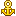使用 gen.coroutine 异步编程

asynchronous 装饰器是让请求变成长连接的方式，必须手动调用 self.finish() 才会响应

def get(self):
self.write("Hello, world")

asynchronous 装饰器不会自动调用self.finish() ，如果没有没有指定结束，该长连接会一直保持直到 pending 状态。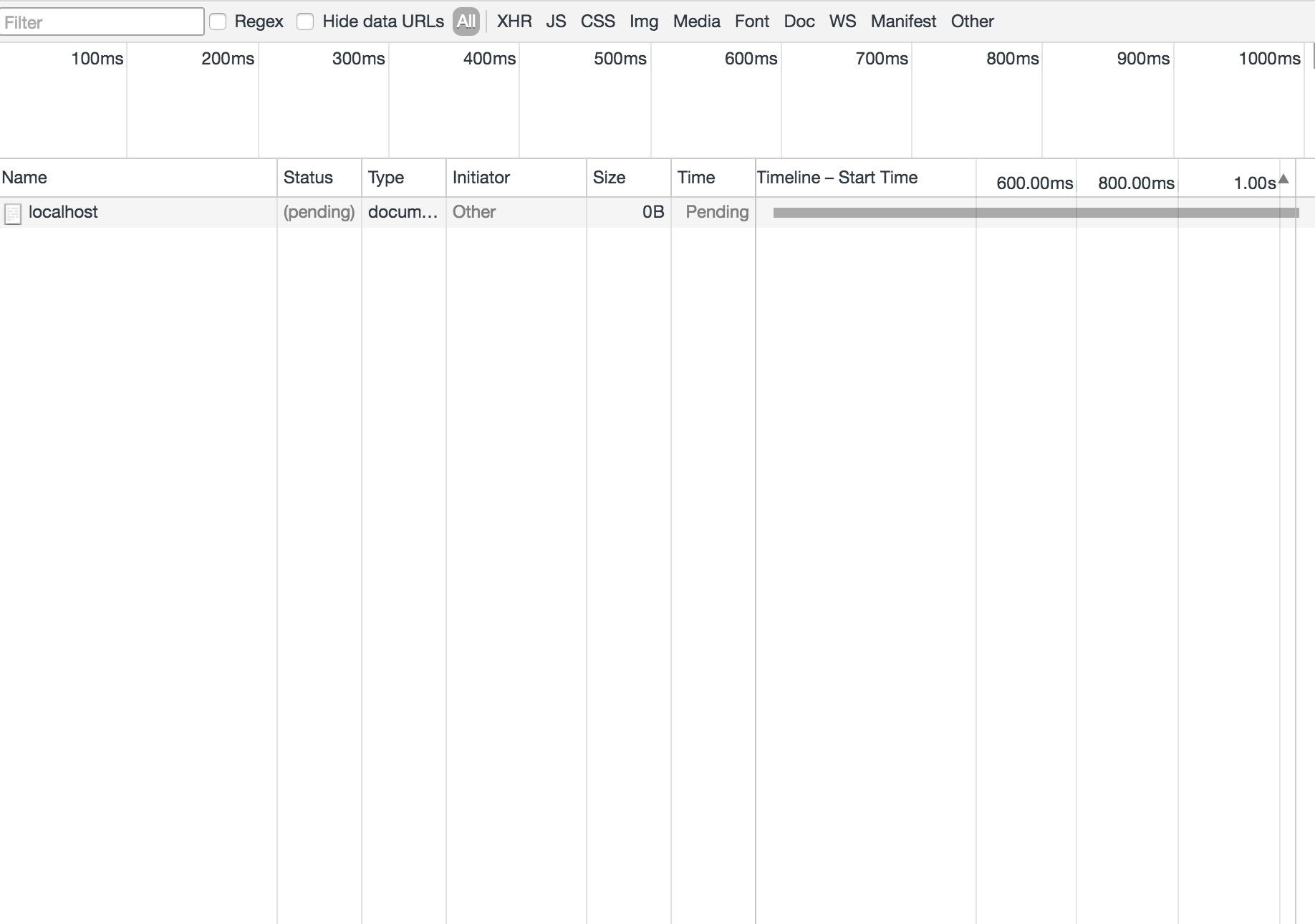def get(self):
self.write("Hello, world")
self.finish()
coroutine 装饰器是指定改请求为协程模式，说明白点就是能使用 yield 配合 Tornado 编写异步程序。

Tronado 为协程实现了一套自己的协议，不能使用 Python 普通的生成器。

@gen.coroutine
def sleep(self):
yield gen.sleep(10)
raise gen.Return([1, 2, 3, 4, 5])

• 需要使用 coroutine 装饰器

• 返回值需要使用 raise
gen.Return()

当做异常抛出

3.2

import time
import logging
from tornado import gen

def get(self):
self.write(“Hello, world”)
self.finish()

@gen.coroutine
def get(self):
yield gen.sleep(10)
self.write(‘Blocking Request’)

def get(self):
time.sleep(10)
self.write(‘Blocking Request’)

def make_app():
(r”/”, MainHandler),

if __name__ == “__main__”:
app = make_app()
app.listen(8000)

gen.sleep(10)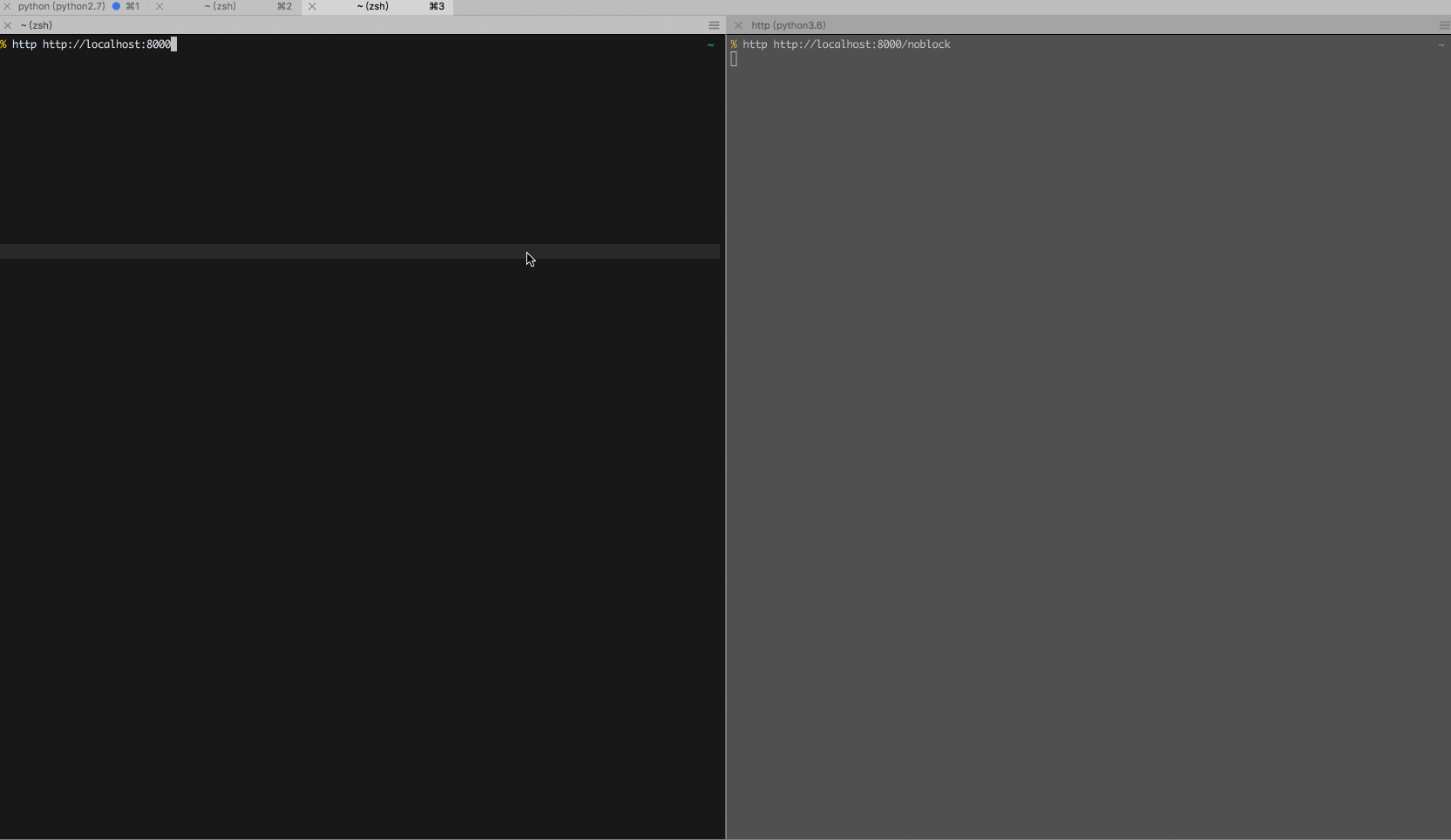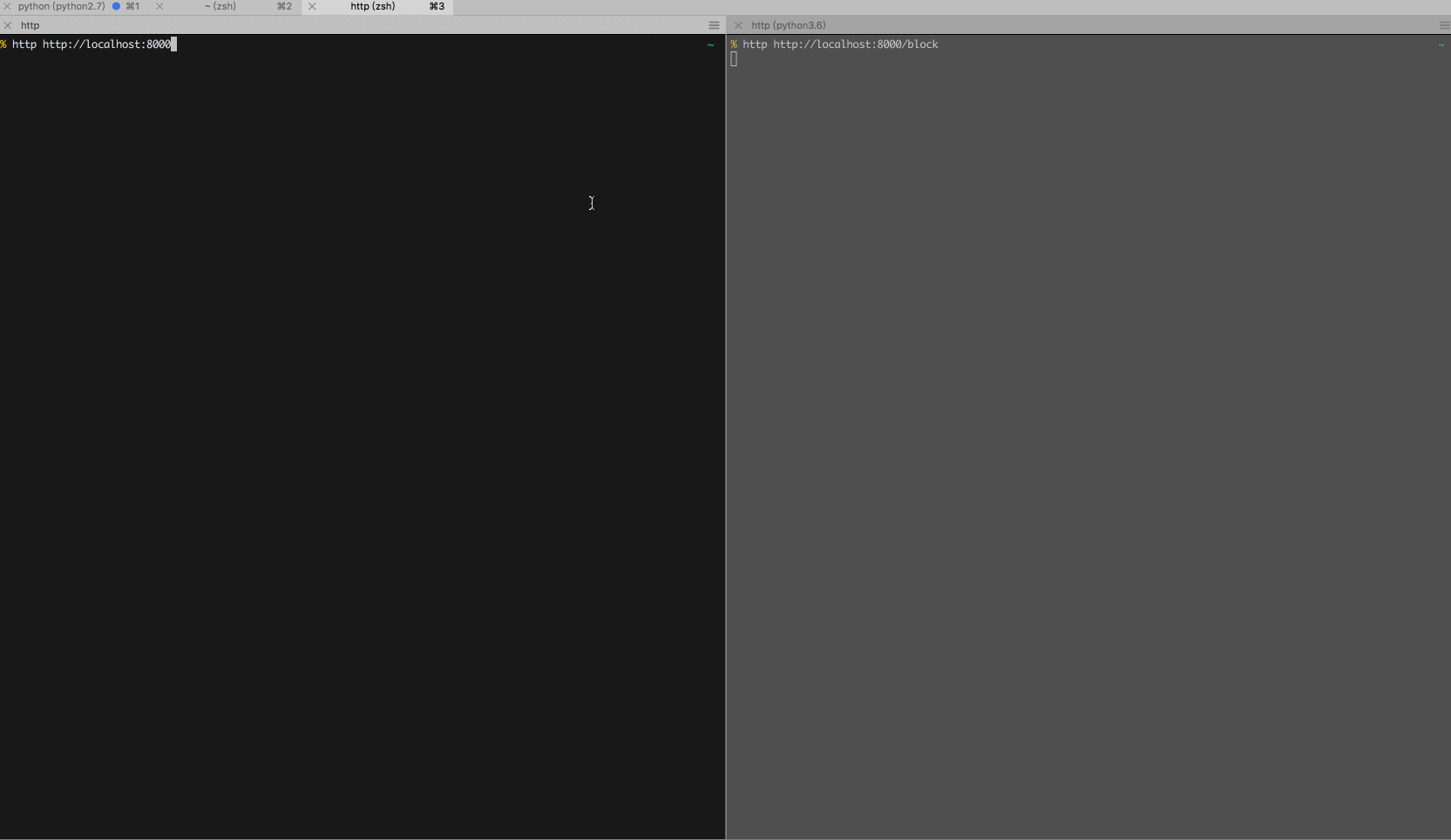3.1基于线程的异步编程

futures Python3 是标准库，但是在 Python2 中需要手动安装
pip
install futures

import logging
from tornado import gen
from tornado.concurrent import run_on_executor
from concurrent.futures import ThreadPoolExecutor

def get(self):
self.write(“Hello, world”)
self.finish()

@run_on_executor
def sleep(self, second):
time.sleep(second)
return second

@gen.coroutine
def get(self):
second = yield self.sleep(5)
self.write(‘noBlocking Request: {}’.format(second))

def make_app():
(r”/”, MainHandler),

if __name__ == “__main__”:
app = make_app()
app.listen(8000)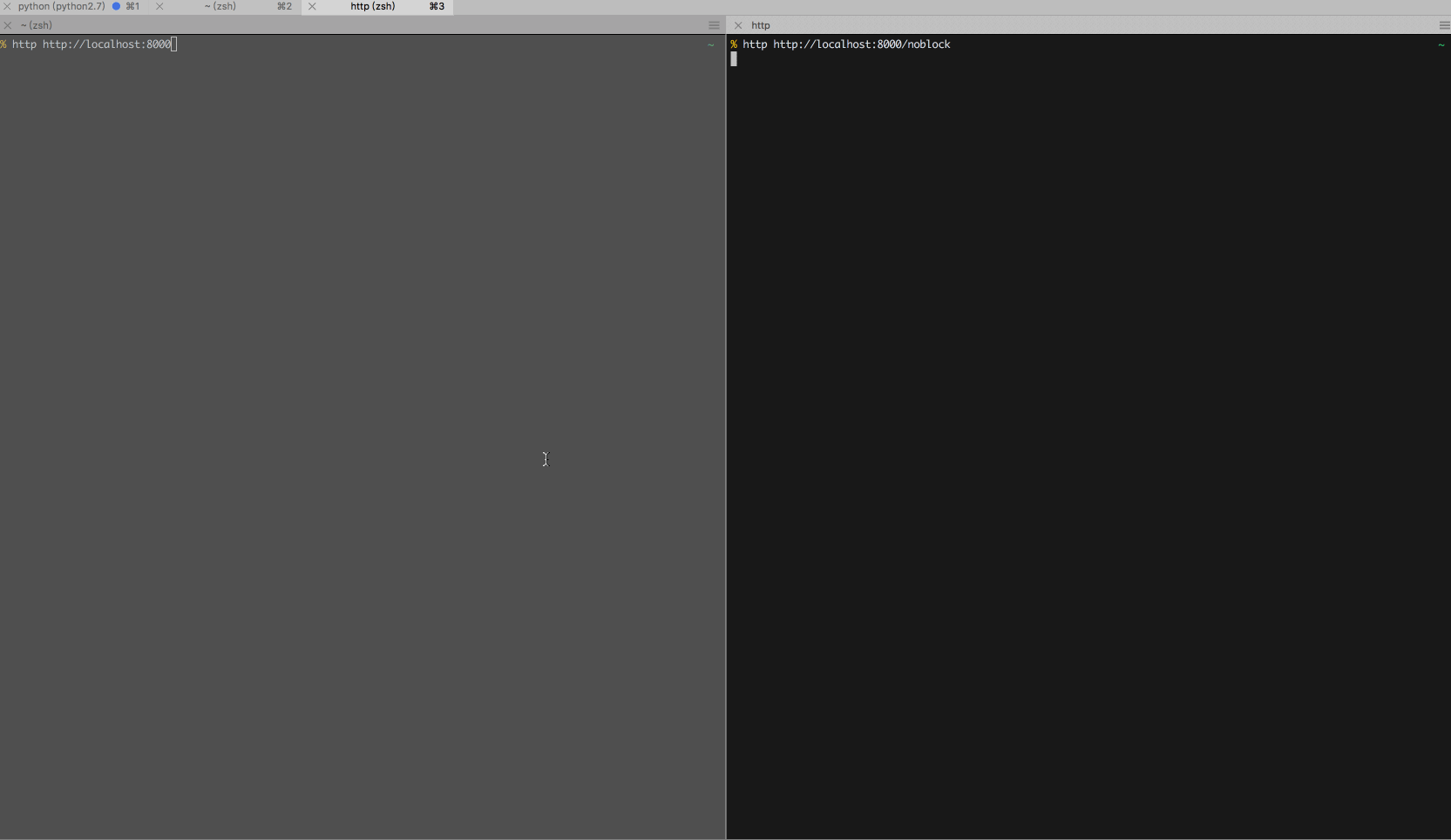基于 Celery 的异步编程

Celery 是一个简单、灵活且可靠的，处理大量消息的分布式系统，专注于实时处理的任务队列，同时也支持任务调度。
Celery

Web

celery 降级到 3.1
pip install celery==3.1
pika 降级到 0.9.14
pip install pika==0.9.14

import time
import logging
from tornado import gen

tcelery.setup_nonblocking_producer()

def get(self):
self.write("Hello, world")
self.finish()

@gen.coroutine
def get(self):
self.write('CeleryBlocking Request: {}'.format(response.result))

def make_app():
(r"/", MainHandler),
(r"/celery-block", CeleryHandler),

if __name__ == "__main__":
app = make_app()
app.listen(8000)
import os
import time
from celery import Celery
from tornado import gen

celery = Celery("tasks", broker="amqp://")
celery.conf.CELERY_RESULT_BACKEND = os.environ.get('CELERY_RESULT_BACKEND', 'amqp')

def sleep(seconds):
time.sleep(float(seconds))
return seconds

if __name__ == "__main__":
celery.start()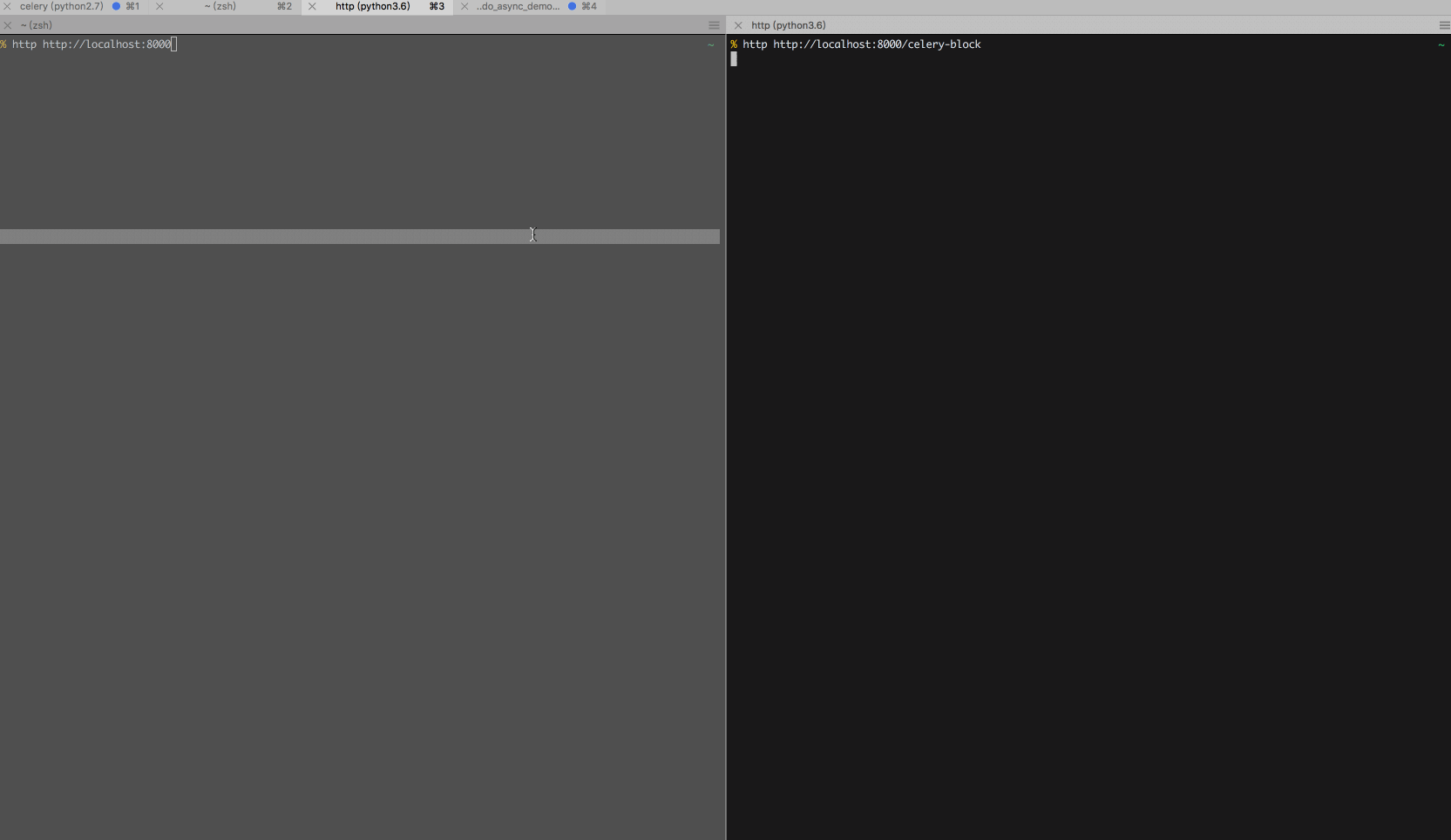Celery Worker 运行在另一个进程中，独立于 Tornado 进程，不会影响 Tornado 运行效率，在处理复杂任务时候比进程模式更有效率。总结

gen.coroutine

★★☆☆☆

★★★☆☆

Celery

★★★☆☆

Python
3

asyncio 模式的库，这应该是日后的方向。Reference

celery  [‘selərɪ]  详细X

n. [园艺] 芹菜

Celery: 芹菜
celery salt: 香芹盐

matplotlib 是 python 最著名的绘图库，它提供了一整套和 matlab 相似的命令 API，十分适合交互式进行绘图。image

代码实现

import matplotlib.pyplot as plt import numpy as np # 定义 x 变量的范围 (-3，3) 数量 50  x=np.linspace(-3,3,50)
y=x**2 # Figure 并指定大小 plt.figure(num=3,figsize=(8,5)) # 绘制 y=x^2 的图像，设置 color 为 red，线宽度是 1，线的样式是 -- plt.plot(x,y,color='red',linewidth=1.0,linestyle='--') # 设置 x，y 轴的范围以及 label 标注 plt.xlim(-1,2)
plt.ylim(-2,3)
plt.xlabel('x')
plt.ylabel('y') # 设置坐标轴刻度线  # Tick X 范围 (-1，2) Tick Label(-1，-0.25，0.5，1.25，2) 刻度数量 5 个 new_ticks=np.linspace(-1,2,5)
plt.xticks(new_ticks) # Tick Y 范围(-2.2,-1,1,1.5,2.4) ，Tick Label (-2.2, -1, 1, 1.5, 2.4) 别名(下面的英文) plt.yticks([-2.2,-1,1,1.5,2.4],
[r'\$really\ bad\$',r'\$bad\$',r'\$normal\$',r'\$good\$',r'\$really\ good\$']) # 设置坐标轴 gca() 获取坐标轴信息 ax=plt.gca() # 使用.spines设置边框：x轴；将右边颜色设置为 none。 # 使用.set_position设置边框位置：y=0的位置；（位置所有属性：outward，axes，data） ax.spines['right'].set_color('none')
ax.spines['top'].set_color('none') # 移动坐标轴 # 将 bottom 即是 x 坐标轴设置到 y=0 的位置。 ax.xaxis.set_ticks_position('bottom')
ax.spines['bottom'].set_position(('data',0)) # 将 left 即是 y 坐标轴设置到 x=0 的位置。 ax.yaxis.set_ticks_position('left')
ax.spines['left'].set_position(('data',0)) # 设置标签 ax.set_title('y = x^2',fontsize=14,color='r') # 显示图像 plt.show()image

基本构成imageimage

Matplotlib 绘图步骤分析image

一、matplotlib 实现简单图像image

#导入 module import matplotlib.pyplot as plt import numpy as np

# 定义 x 变量的范围 (-3，3) 数量 50  x=np.linspace(-3,3,50)
y=x**2

#绘制及显示 plt.figure()
plt.plot(x, y)
plt.show()

import matplotlib.pyplot as plt import numpy as np # 定义 x 变量的范围 (-3，3) 数量 50  x=np.linspace(-3,3,50)
y=x**2 plt.figure()
plt.plot(x, y)
plt.show()image

二、设置 Figure 图像

plt.figure(num=3,figsize=(8,5))
plt.plot(x,y,color='red',linewidth=1.0,linestyle='--')

import matplotlib.pyplot as plt import numpy as np # 定义 x 变量的范围 (-3，3) 数量 50  x=np.linspace(-3,3,50)
y=x**2 # Figure 并指定大小 plt.figure(num=3,figsize=(8,5)) # 绘制 y=x^2 的图像，设置 color 为 red，线宽度是 1，线的样式是 -- plt.plot(x,y,color='red',linewidth=1.0,linestyle='--')

plt.show()image

三、设置坐标轴

设置 x，y 轴坐标范围及标注 label

import matplotlib.pyplot as plt import numpy as np # 定义 x 变量的范围 (-3，3) 数量 50  x=np.linspace(-3,3,50)
y=x**2 # Figure 并指定大小 plt.figure(num=3,figsize=(8,5)) # 绘制 y=x^2 的图像，设置 color 为 red，线宽度是 1，线的样式是 -- plt.plot(x,y,color='red',linewidth=1.0,linestyle='--') # 设置 x，y 轴的范围以及 label 标注 plt.xlim(-1,2)
plt.ylim(-2,3)
plt.xlabel('x')
plt.ylabel('y')

plt.show()image

设置 x, y 刻度范围及刻度标注

# 设置坐标轴刻度线  # Tick X 范围 (-1，2) Tick Label(-1，-0.25，0.5，1.25，2) 刻度数量 5 个 new_ticks=np.linspace(-1,2,5)
plt.xticks(new_ticks) # Tick Y 范围(-2.2,-1,1,1.5,2.4) ，Tick Label (-2.2, -1, 1, 1.5, 2.4) 别名(下面的英文) plt.yticks([-2.2,-1,1,1.5,2.4],

import matplotlib.pyplot as plt import numpy as np # 定义 x 变量的范围 (-3，3) 数量 50  x=np.linspace(-3,3,50)
y=x**2 # Figure 并指定大小 plt.figure(num=3,figsize=(8,5)) # 绘制 y=x^2 的图像，设置 color 为 red，线宽度是 1，线的样式是 -- plt.plot(x,y,color='red',linewidth=1.0,linestyle='--') # 设置 x，y 轴的范围以及 label 标注 plt.xlim(-1,2)
plt.ylim(-2,3)
plt.xlabel('x')
plt.ylabel('y') # 设置坐标轴刻度线  # Tick X 范围 (-1，2) Tick Label(-1，-0.25，0.5，1.25，2) 刻度数量 5 个 new_ticks=np.linspace(-1,2,5)
plt.xticks(new_ticks) # Tick Y 范围(-2.2,-1,1,1.5,2.4) ，Tick Label (-2.2, -1, 1, 1.5, 2.4) 别名(下面的英文) plt.yticks([-2.2,-1,1,1.5,2.4],image

四、移动坐标轴

1. 使用plt.gca获取当前坐标轴信息。

2. 使用.spines设置边框：右侧边框；使用.set_color设置边框颜色：默认白色；

使用.spines设置边框：上边框；使用.set_color设置边框颜色：默认白色；

1. 使用.xaxis.set_ticks_position设置 x 坐标刻度数字或名称的位置：bottom。（所有位置：top，bottom，both，default，none）。
2. 使用.spines设置边框：x 轴；使用.set_position设置边框位置：y=0 的位置；（位置所有属性：outward，axes，data）
# 设置坐标轴 gca() 获取坐标轴信息 ax=plt.gca() # 使用.spines设置边框：x轴；将右边颜色设置为 none。 # 使用.set_position设置边框位置：y=0的位置；（位置所有属性：outward，axes，data） ax.spines['right'].set_color('none')
ax.spines['top'].set_color('none') # 移动坐标轴 # 将 bottom 即是 x 坐标轴设置到 y=0 的位置。 ax.xaxis.set_ticks_position('bottom')
ax.spines['bottom'].set_position(('data',0)) # 将 left 即是 y 坐标轴设置到 x=0 的位置。 ax.yaxis.set_ticks_position('left')
ax.spines['left'].set_position(('data',0))

import matplotlib.pyplot as plt import numpy as np # 定义 x 变量的范围 (-3，3) 数量 50  x=np.linspace(-3,3,50)
y=x**2 # Figure 并指定大小 plt.figure(num=3,figsize=(8,5)) # 绘制 y=x^2 的图像，设置 color 为 red，线宽度是 1，线的样式是 -- plt.plot(x,y,color='red',linewidth=1.0,linestyle='--') # 设置 x，y 轴的范围以及 label 标注 plt.xlim(-1,2)
plt.ylim(-2,3)
plt.xlabel('x')
plt.ylabel('y') # 设置坐标轴刻度线  # Tick X 范围 (-1，2) Tick Label(-1，-0.25，0.5，1.25，2) 刻度数量 5 个 new_ticks=np.linspace(-1,2,5)
plt.xticks(new_ticks) # Tick Y 范围(-2.2,-1,1,1.5,2.4) ，Tick Label (-2.2, -1, 1, 1.5, 2.4) 别名(下面的英文) plt.yticks([-2.2,-1,1,1.5,2.4],
[r'\$really\ bad\$',r'\$bad\$',r'\$normal\$',r'\$good\$',r'\$really\ good\$']) # 设置坐标轴 gca() 获取坐标轴信息 ax=plt.gca() # 使用.spines设置边框：x轴；将右边颜色设置为 none。 # 使用.set_position设置边框位置：y=0的位置；（位置所有属性：outward，axes，data） ax.spines['right'].set_color('none')
ax.spines['top'].set_color('none') # 移动坐标轴 # 将 bottom 即是 x 坐标轴设置到 y=0 的位置。 ax.xaxis.set_ticks_position('bottom')
ax.spines['bottom'].set_position(('data',0)) # 将 left 即是 y 坐标轴设置到 x=0 的位置。 ax.yaxis.set_ticks_position('left')
ax.spines['left'].set_position(('data',0)) # 显示图像 plt.show()image

五、设置标题

# 设置标签 ax.set_title('y = x^2',fontsize=14,color='r')

import matplotlib.pyplot as plt import numpy as np # 定义 x 变量的范围 (-3，3) 数量 50  x=np.linspace(-3,3,50)
y=x**2 # Figure 并指定大小 plt.figure(num=3,figsize=(8,5)) # 绘制 y=x^2 的图像，设置 color 为 red，线宽度是 1，线的样式是 -- plt.plot(x,y,color='red',linewidth=1.0,linestyle='--') # 设置 x，y 轴的范围以及 label 标注 plt.xlim(-1,2)
plt.ylim(-2,3)
plt.xlabel('x')
plt.ylabel('y') # 设置坐标轴刻度线  # Tick X 范围 (-1，2) Tick Label(-1，-0.25，0.5，1.25，2) 刻度数量 5 个 new_ticks=np.linspace(-1,2,5)
plt.xticks(new_ticks) # Tick Y 范围(-2.2,-1,1,1.5,2.4) ，Tick Label (-2.2, -1, 1, 1.5, 2.4) 别名(下面的英文) plt.yticks([-2.2,-1,1,1.5,2.4],
[r'\$really\ bad\$',r'\$bad\$',r'\$normal\$',r'\$good\$',r'\$really\ good\$']) # 设置坐标轴 gca() 获取坐标轴信息 ax=plt.gca() # 使用.spines设置边框：x轴；将右边颜色设置为 none。 # 使用.set_position设置边框位置：y=0的位置；（位置所有属性：outward，axes，data） ax.spines['right'].set_color('none')
ax.spines['top'].set_color('none') # 移动坐标轴 # 将 bottom 即是 x 坐标轴设置到 y=0 的位置。 ax.xaxis.set_ticks_position('bottom')
ax.spines['bottom'].set_position(('data',0)) # 将 left 即是 y 坐标轴设置到 x=0 的位置。 ax.yaxis.set_ticks_position('left')
ax.spines['left'].set_position(('data',0)) # 设置标签 ax.set_title('y = x^2',fontsize=14,color='r') # 显示图像 plt.show()image